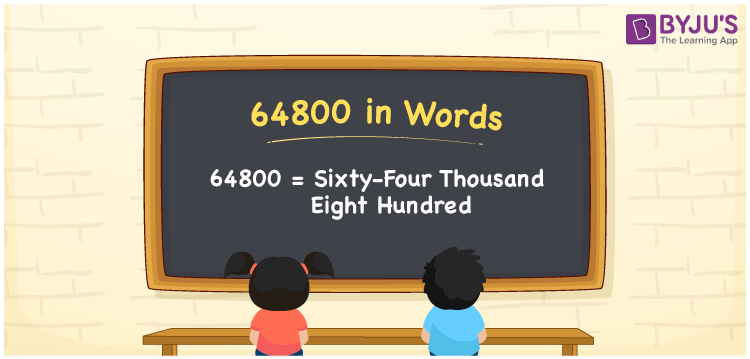# 64800 in Words

Sixty-four thousand eight hundred is the number name for the numeral 64800. For example, if you saved Rs. 64800 in 7 months, you can write it as “I saved Rs. Sixty-four thousand eight hundred in seven months”. Generally, for converting the numbers into words, we will use the place value system. In this article, let us learn how to spell the number 64800 in words with a complete explanation.

 64800 in Words: Sixty-four Thousand Eight Hundred. Sixty-four Thousand Eight Hundred in Numerical Form: 64800.

## 64800 in English Words## How to Write 64800 in Words?

Below table depicts the place values of 64800:

 Ten-thousands Thousands Hundreds Tens Ones 6 4 8 0 0

The expanded form of 64800 is as follows:

= 6 × Ten thousand + 4 × Thousand + 8 × Hundred + 0 × Ten + 0 × One

= 6 × 10000 + 4 × 1000 + 8 × 100 + 0 × 10 + 0 × 1

= 60000 + 4000 + 800

= 64800

= Sixty-four thousand eight hundred

Hence, 64800 in words is sixty-four thousand eight hundred.

64800 in words – Sixty-four thousand eight hundred

Is 64800 an odd number? – No

Is 64800 an even number? – Yes

Is 64800 a perfect square number? – No

Is 64800 a perfect cube number? – No

Is 64800 a prime number? – No

Is 64800 a composite number? – Yes

## Frequently Asked Questions on 64800 in Words

Q1

### How to write 64800 in words?

64800 in words is sixty-four thousand eight hundred.

Q2

### Simplify 64000 + 800, and express it in words.

Simplifying 64000 + 800, we get 64800. Hence, 64800 in words is sixty-four thousand eight hundred.

Q3

### Is 64800 an even number?

Yes, 64800 is an even number.